## Convert date to month and day only in excel

In excel, how to get the month and day string from an excel date value. How to convert the dates to text string, just only display as month and day. This post will guide you how to convert date to month and day value only using Text Function in Microsoft Excel.

## Convert date to month and day with Text Function

If you want to convert the date (mm/dd/yyyy) to month and day only, you can use “mm dd” format code within the TEXT function in excel, so you can write down the below TEXT formula:

`=TEXT(date,"mm dd")`

Assuming that you want to get the text string of month and year only from the date value: 12/1/2018 in Cell B1, then you can add another column and use the below formula in Cell C1 like:

`=TEXT(B1,"mm dd")`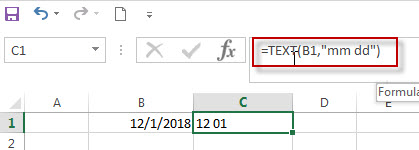If you are using the format code as “mmmm dd”, then the full name of month will display in Cell C1.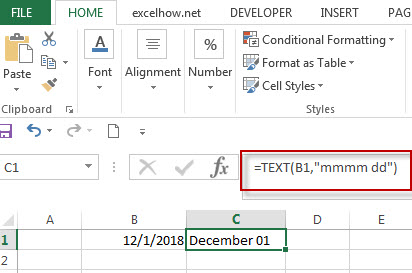For more format codes in excel formatting, you can refer to the below table:

 Format Code Description Examples 0 only display digits in its place #.00 – Forces the function to display two decimal places =Text(34.234,”\$##.00″) result: \$34.23 # Display the placeholder =Text(4.527,”#.##) result: 4.53 . the position of Decimal Point =Text(342.2,”0.00″) result: 342.20 d Day of the month or day of week d- one or two digit number (1-31) dd- two digit number (01-31) ddd-abbreviated day of week (Mon to Sun) dddd-full name of day of week(Monday to Sunnday) =Text(TODAY(),”DDDD”) result: Monday m The Month of the Year m- one or two digit number mm-two digit number mmm-abbreviated month(Jan to Dec) mmmm-full name of month(January to December)) =Text(TODAY(),”MM/DD/YY”) result:11/06/17 y year yy-two digit representation of year(e.g.01,17) yyyy-four digit representation of year(e.g. 2001,2017) =Text(TODAY(),”MM/DD/YY”) result:11/06/17

### Related Formulas

• Excel Convert numbers to Text
The Text function will accept a numeric value as the first argument, then based on the format code in the second argument to convert the number to text. You can convert all the standard number formats such as: dates, times, currency to Text string in excel.…
• Convert date to month name with Text Function
If you want to convert the date to a month name, you can use the TEXT function with a specified format code like “mmm”in excel.  You can try to run the following TEXT formula:=TEXT(B1,”mmm”)
• Convert Date to text with Text Function in Excel
you can use TEXT function in excel to convert dates to text in a specific format code. For example, The TEXT function can use the following patterns, like as: “mm/dd/yyyy”, “yyyy/mm/dd”, etc.
• Convert date to month and year only in excel
If you want to convert the date to month and year only, you can use “yyyymm” format code within the TEXT function in excel, so you can write down the below TEXT formula:=TEXT(date,”yyyymm”)

### Related Functions

• Excel Text function
The Excel TEXT function converts a numeric value into text string with a specified format. The TEXT function is a build-in function in Microsoft Excel and it is categorized as a Text Function. The syntax of the TEXT function is as below: = TEXT (value, Format code)…

## Three Ways to Convert Number to Text in Excel

Before we have wrote a post to describe that how to convert number to Text string with Text Function in excel. This post will guide you how to convert number to Text using The Format Cells Command and Text to Columns wizard in excel.

## Convert Number to Text Using Format Cells Option

You also can be used the Format Cells option to change the number to Text string in excel.  It may be another quick way to change the numeric value to text string in excel.

1# select the range with the numbers that you want to convert to text.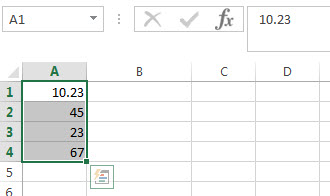2#Right-click the selected range in the step1, and then click “Format Cells...” item from the pop-up menu list.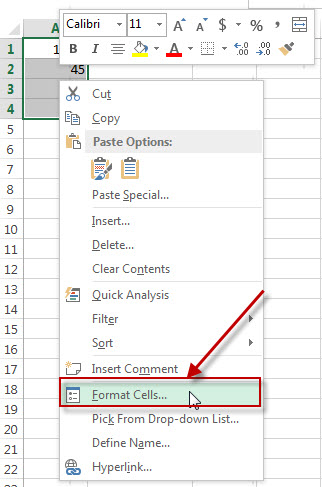3# Format Cells” window will appear.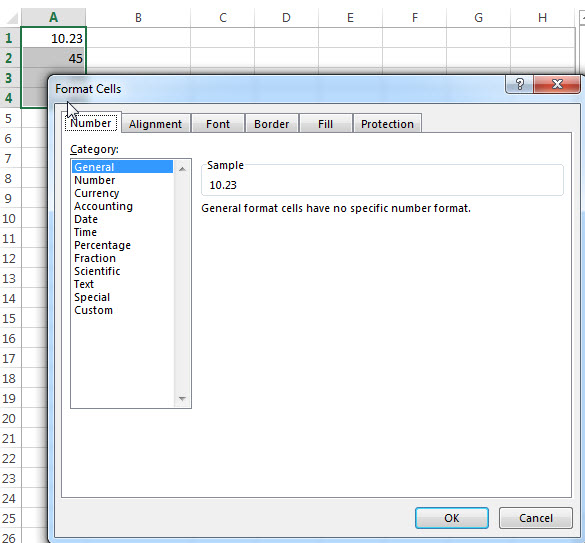4# Select “Text” under the Number tab and then click OK button.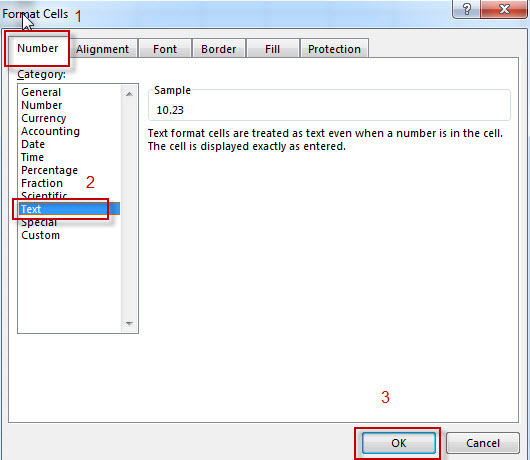## Convert Number to Text Using Text to Columns wizard

1# Select the column that you want to convert numbers to text string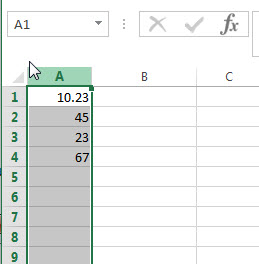2# click on the “Text to Columns” button under DATA tab on excel ribbon.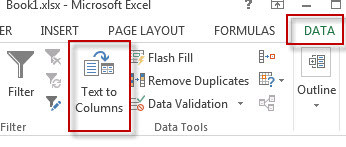3#Convert Text to Columns Wizard” window will appear.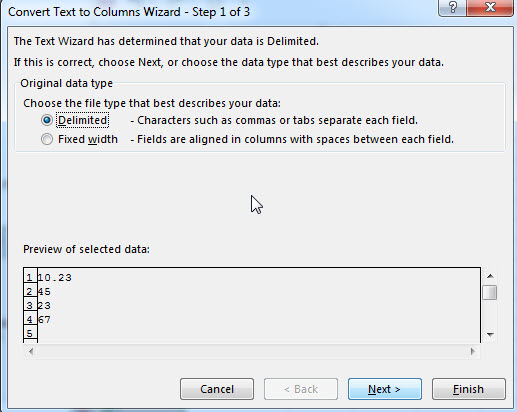4# click “next” button through step 1 and step 2. Select “Text” radio button under step 3. Then click on “Finish” button.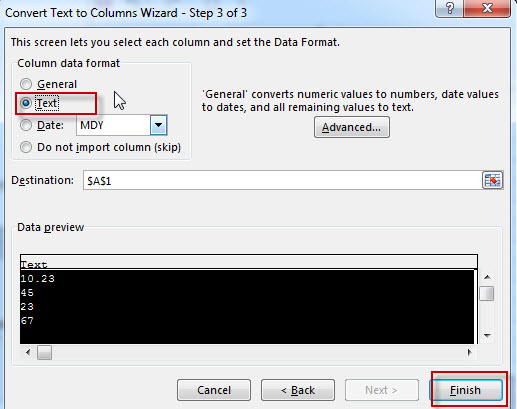You will see that the numbers is converted to text now.

## Convert Number to Text Using Concatenate Operator

We can also use another way to change the numeric value to string using Excel Concatenate operator.

The concatenation operator (&) can join together text and numbers into a single text string in excel. So if you just concatenate an empty text string (such as: “”) and a numeric value together, the returned value will be a text string. Let’s see the below example:

`=""&A1`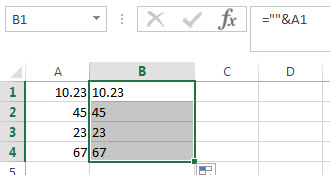You need to enter the above excel formula in the B1 cell, then click “enter”. You will see that the numeric value in Cell A1 be changed as text string in B1.

Also read: Excel Convert numbers to Text

## Excel Convert numbers to Text

This post will guide you how to convert numbers to text with the Excel TEXT function. Assuming that you want to display leading Zero in numbers in cell, then you need to convert them into Text based on the specified Format_text. So you can use TEXT function to achieve this in Excel.

The Text function will accept a numeric value as the first argument, then based on the format code in the second argument to convert the number to text. You can convert all the standard number formats such as: dates, times, currency to Text string in excel.

## Convert Number to Text with Text Function

If you just want to convert the numbers in the cell to Text without any formatting, then you can use the below generic formula of Text function in excel:

`=Text(B1,"0")`

​In the above formula, it will convert the number to text string with no special formatting, as we just use the most simply format text as “0“.

If you want to display the leading zero in numbers, then you can use the format_text as “0000” in the Excel Text function as follows:

`=Text(B1,"0000")`

If you want to convert the number in cell B1 to text string which keep three decimal digits, the following format text can be used in the Excel Text function:

00.000

we can write down the formula using Text function in excel as follows:

`=Text(B1,"00.000")`

For more format codes in excel formatting, you can refer to the below table:

 Format Code Description Examples 0 only display digits in its place #.00 – Forces the function to display two decimal places =Text(34.234,”\$##.00″) result: \$34.23 # Display the placeholder =Text(4.527,”#.##) result: 4.53 . the position of Decimal Point =Text(342.2,”0.00″) result: 342.20 d Day of the month or day of week d- one or two digit number (1-31) dd- two digit number (01-31) ddd-abbreviated day of week (Mon to Sun) dddd-full name of day of week(Monday to Sunnday) =Text(TODAY(),”DDDD”) result: Monday m The Month of the Year m- one or two digit number mm-two digit number mmm-abbreviated month(Jan to Dec) mmmm-full name of month(January to December)) =Text(TODAY(),”MM/DD/YY”) result:11/06/17 y year yy-two digit representation of year(e.g.01,17) yyyy-four digit representation of year(e.g. 2001,2017) =Text(TODAY(),”MM/DD/YY”) result:11/06/17 h Hour h-one or two digit number (e.g. 1,23) hh-two digit number (e.g. 01,23) =Text(14:16,”hh:mm”) result: 14:16 m Minute m-one or two digit representation (e.g. 1,59) mm-two digit representation (e.g. 01,59) =Text(14:16,”hh:mm”) result: 14:16 s Second s-one or two digit representation (e.g. 1,59) ss=two digit representation (e.g. 01,59)

### Related Functions

• Excel Text function
The Excel TEXT function converts a numeric value into text string with a specified format. The TEXT function is a build-in function in Microsoft Excel and it is categorized as a Text Function. The syntax of the TEXT function is as below: = TEXT (value, Format code)…